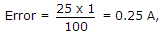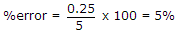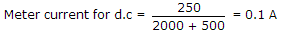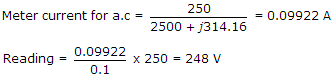# Electronics and Communication Engineering - Measurements and Instrumentation

1.
To increase Q factor of a coil, the wire should be
long
thin
thick
long and thin
Explanation:

Q is high if resistance is low. Therefore, wire should be thick.

2.
An ammeter of 0-25 A range has a guaranteed accuracy of 1% of full scale reading. The current measured is 5 A. The limiting error is
2%
2.5%
4%
5%
Explanation:.

3.
The coil of a moving iron instrument has a resistance of 500 Ω and an inductance of 1 H. It reads 250 V when a 250 V dc is applied. If series resistance is 2000 Ω, its reading when fed by 250 V, 50 Hz ac will be
260 V
252 V
250 V
248 V
Explanation:4.
In 3 phase power measurement by two wattmeter method, the reading of one wattmeter is zero. The power factor of load is
1
0.5
0
0.8
Explanation:

When p.f. = 0.5, 0 = 60° and one of the wattmeters reads zero.

5.
In a CRO which of the following is not a part of electron gun
cathode
grid
accelerating anode
X - Y plates